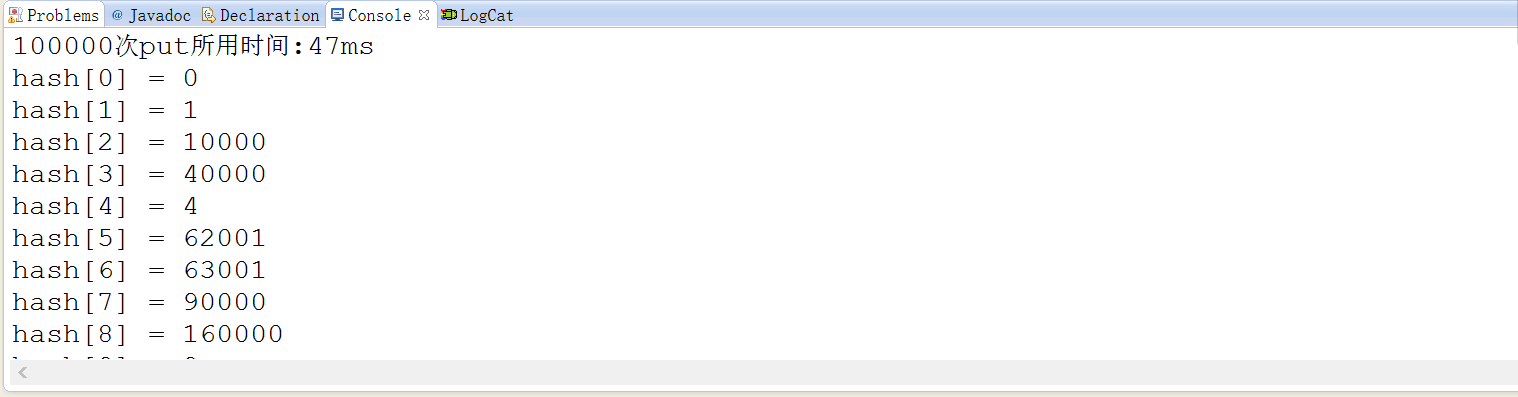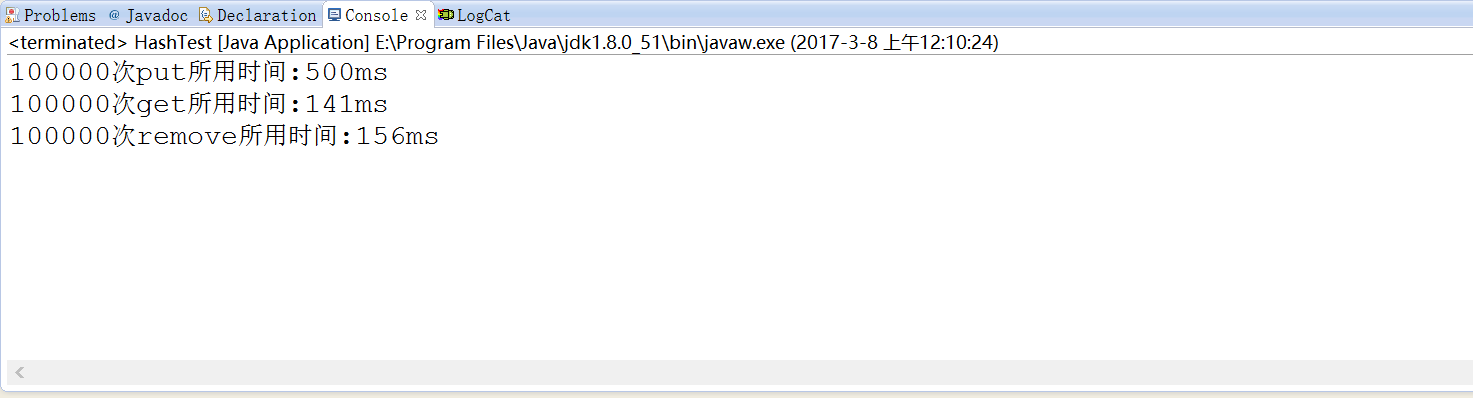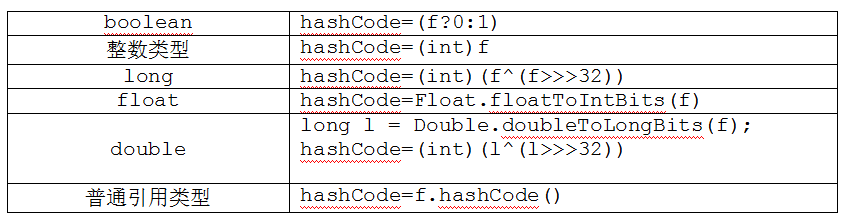# Java中的hashmap``````所谓哈希表，是一种数据的存储结构，我们可以通过数据的“关键码值”来直接访问表。通过散列的方法(可以理解为一种特殊的数学过程=_=)，我们可以把关键码值映射到数组中的不同位置。

``````public class HashDemo {

static int size = 1000;
static int[] hash = new int[size];
int currsize = 0;
int Key, Value;

static {
for (int i = 0; i < size; i++) {
hash[i] = -1;
}
}

// 构造方法
public HashDemo(int Key, int Value) {
this.Key = Key;
this.Value = Value;
}

public HashDemo() {}

// 计算hashcode
public int hashcode(int Key) {
int index = Key % size;
if (currsize == size) {
return -1;
} else {
while (index >= 0 && index < size && hash[index] != -1) {
index++;
if (index == size - 1) {
index++;
}
}
return index;
}
}

// 往HashDemo中插入测试数据
public boolean put(int Key, int Value) {
int index = hashcode(Key);
if (index == -1 || index < 0 || index >= size)
return false;
else {
hash[index] = Value;
return true;
}
}

public void Tester() {
HashDemo hd = new HashDemo();
long starttime = System.currentTimeMillis();

for (int i = 0; i < 100000; i++) {
hd.put(i * i, i * i);
}
long endtime = System.currentTimeMillis();
System.out.println("100000次put所用时间:" + (endtime - starttime) + "ms");
}

}````````````import java.util.HashMap;

public class HashTest {

public void Tester(){
HashMap<Integer,Integer> hm=new HashMap<Integer,Integer>();

long starttime=System.currentTimeMillis();

for(int i=0;i<1000000;i++){
Integer n=new Integer(i);
hm.put(n*n,n*n );
}

long endtime=System.currentTimeMillis();
System.out.println("100000次put所用时间:"+(endtime-starttime)+"ms");

starttime=System.currentTimeMillis();

for(int i=0;i<1000000;i++){
Integer n=new Integer(i);
Integer m=hm.get(n*n);
}

endtime=System.currentTimeMillis();
System.out.println("100000次get所用时间:"+(endtime-starttime)+"ms");

starttime=System.currentTimeMillis();

for(int i=0;i<1000000;i++){
Integer n=new Integer(i);
hm.remove(n*n);
}

endtime=System.currentTimeMillis();
System.out.println("100000次remove所用时间:"+(endtime-starttime)+"ms");
}````````````可以看到使用java中自带的hashmap类执行put操作时，和上述思路所花费的时间有较大的出入，并且可以发现多次put和get操作所花费的时间也有较大的差别。

``````
``````

![img](https://img-blog.csdn.net/20170308230629818?watermark/2/text/aHR0cDovL2Jsb2cuY3Nkbi5uZXQvSE5VX0NzZWVfd2p3/font/5a6L5L2T/fontsize/400/fill/I0JBQkFCMA==/dissolve/70/gravity/SouthEast)
``````

``````

``````

``````        ①同一个对象调用hashCode()时，应返回相同的值
②若两个对象通过equals()方法比较返回true的时候，两个对象的hashCode()方法应返回相同的值
③对象调用equals()方法参与比较的值，都应该拿来计算hashCode()
````````将所有的数据计算出来的hashCode相加即为该对象的hashCode();为了避免重复，可以让各个数据乘以不同的权值后再相加。``

-- 展开阅读全文 --

« 上一篇 07-24